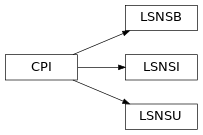# 5.9. pyopus.problems.karmitsa — Large scale nonsmooth test functions (Karmitsa set)¶Large scale nonsmooth test functions (Karmitsa set) (PyOPUS subsystem name: KARMITSA)

This module is independent of PyOPUS, meaning that it can be taken as is and used as a module in some other package. It depends only on the cpi and the _karmitsa modules.

karmitsa

Karmitsa N.: Test Problems for Large-Scale Nonsmooth Minimization, Technical report B.4/2007, University of Jyvaskyla, Jyvaskyla, 2007.

class pyopus.problems.karmitsa.LSNSB(name=None, number=None, n=2, feasible=1)[source]

Bound constrained problems from the Karmitsa test suite.

• name - problem name

• number - problem number (0-9)

• n - problem dimension

• feasible - 0=initial point unchanged, 1=feasible, 2=strictly feasible, default=1

Attributes:

• name - problem name

• n - number of variables

• xl - lower bounds on variables

• xh - upper bounds on variables

• initial - initial values of variables

• fmin - best known minimum

This module is independent of PyOPUS, meaning that it can be taken as is and used as a module in some other package. It depends only on the cpi and the _karmitsa modules.

cpi()[source]

Returns the common problem interface.

Subgradient is not supported.

xmin is also not available.

See the CPI class for more information.

f(x)[source]

Returns the value of the function at x.

names = ['GeneralizedMAXQ', 'GeneralizedMXHILB', 'ChainedLQ', 'ChainedCB3I', 'ChainedCB3II', 'ActiveFaces', 'GeneralizedBrown2', 'ChainedMifflin2', 'ChainedCrescentI', 'ChainedCrescentII']

List of all function names

class pyopus.problems.karmitsa.LSNSI(name=None, number=None, cname=None, cnumber=None, n=10)[source]

Inequality constrained problems from the Karmitsa test suite.

• name - function name

• number - function number (0-9)

• cname - constraint name

• cnumber - constraint number (0-7)

• n - problem dimension

Attributes:

• name - problem name

• fname - function name

• cname - constraint name

• n - number of variables

• m - number of constraints

• cl - lower bounds on constraint functions

• ch - upper bounds on constraint functions

• initial - initial values of variables

This module is independent of PyOPUS, meaning that it can be taken as is and used as a module in some other package. It depends only on the cpi and the _karmitsa modules.

c(x)[source]

Returns the value of the constraints at x.

cnames = ['TridiagonalI', 'TridiagonalII', 'MAD1I', 'MAD1II', 'ModifiedMAD1I', 'ModifiedMAD1II', 'P20I', 'P20II']

List of all constraint names

cpi()[source]

Returns the common problem interface.

Subgradient is not supported.

xmin is also not available.

See the CPI class for more information.

f(x)[source]

Returns the value of the function at x.

names = ['GeneralizedMAXQ', 'GeneralizedMXHILB', 'ChainedLQ', 'ChainedCB3I', 'ChainedCB3II', 'ActiveFaces', 'GeneralizedBrown2', 'ChainedMifflin2', 'ChainedCrescentI', 'ChainedCrescentII']

List of all function names

class pyopus.problems.karmitsa.LSNSU(name=None, number=None, n=2)[source]

Unconstrained problems from the Karmitsa test suite. Problems 10 and 11 were added by Á. Bűrmen.

• name - problem name

• number - problem number (0-11)

• n - problem dimension

Attributes:

• name - problem name

• n - number of variables

• initial - initial values of variables

• fmin - best known minimum

This module is independent of PyOPUS, meaning that it can be taken as is and used as a module in some other package. It depends only on the cpi and the _karmitsa modules.

cpi()[source]

Returns the common problem interface.

Subgradient is not supported.

xmin is also not available.

See the CPI class for more information.

f(x)[source]

Returns the value of the function at x.

names = ['GeneralizedMAXQ', 'GeneralizedMXHILB', 'ChainedLQ', 'ChainedCB3I', 'ChainedCB3II', 'ActiveFaces', 'GeneralizedBrown2', 'ChainedMifflin2', 'ChainedCrescentI', 'ChainedCrescentII', 'GeneralizedL1HILB', 'GeneralizedWatson']

List of all function names

Example file karmitsa.py in folder demo/problems/

# Karmitsa test suite

from pyopus.problems.karmitsa import *

if __name__=='__main__':
print("Unconstrained, n=50")
for ii in range(10):
prob=LSNSU(number=ii, n=50)
print("%d: %25s: f0=%e" % (ii, prob.name, prob.f(prob.initial)))
print()

print("Bound constrained, n=50")
for ii in range(10):
prob=LSNSB(number=ii, n=50)
print("%d: %25s: f0=%e" % (ii, prob.name, prob.f(prob.initial)))
print()

print("Inequality constrained, n=10")
for ii in range(10):
for jj in range(8):
prob=LSNSI(number=ii, cnumber=jj, n=10)
c=prob.c(prob.initial)
feas=((c>prob.cl) & (c<prob.ch)).all()
print("%d, %d: %30s: f0=%12.4e feas=%d" % (ii,jj, prob.name, prob.f(prob.initial), feas))
print()